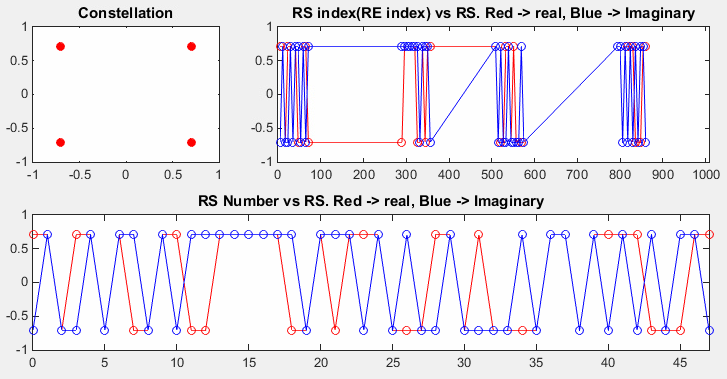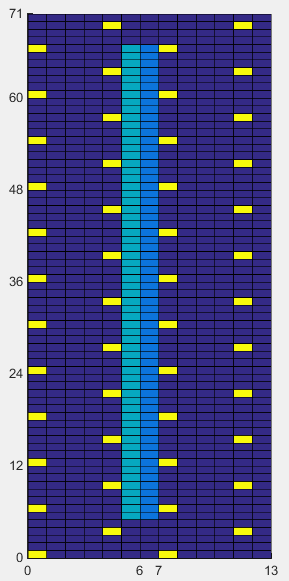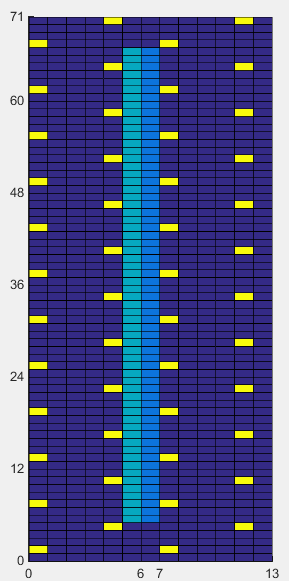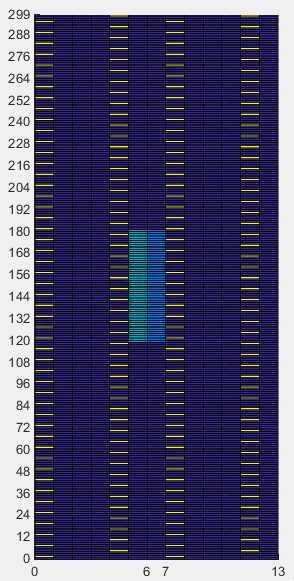MatLab Toolbox - LTE                                      Home : www.sharetechnote.com

Cell Specific Reference Signal

There are many different types of Reference Signal that may confuse a lot of people including me. It is confusing not only in terms of too many different types but also in terms of terminology refering to those reference signal. Refer to Downlink Reference Signal page and have good understanding of various types of Reference Signal. In this example, I will deal with only Cell Specific Reference signal (p0~p3, mostly p0 only for simplicify).

< SISO - RS Symbol Generation >

% Since PSS is determined by each eNodeB, you have to define properites of a eNodeB.

% NDLRB indicate System Bandwith in the unit of RBs.

% NDLRB 6 = 1.4 Mhz, NDLRB 15 = 3.0 Mhz, NDLRB 25 = 5.0 Mhz,

% NDLRB 50 = 10 Mhz, NDLRB 75 = 15 Mhz, NDLRB 100 = 20 Mhz

% CellRefP indicate number of downlink Antenna. CellRefP = 1 means 1 transmission antenna (SISO)

% NCellID indicate PCI (Physical Channel Identity) of the Cell

% NSubframe indicate the subframe number.

enb.CyclicPrefix = 'Normal';

enb.NDLRB = 6;

enb.CellRefP = 1;

enb.DuplexMode = 'FDD';

enb.NCellID = 10;

enb.NSubframe = 1;

% Then pass the eNodeB definition to lteCellRS() with a specific antenna port number,

% it would give you the list of Cell Specific Reference Signal. '0' in lteCellRS(enb,0) means 'antenna port 0'.

% lteCellRSIndices() gives you the list of RE (Resource Element) number within the whole subframe.

rsAnt0 = lteCellRS(enb,0);

indAnt0 = lteCellRSIndices(enb,0);

indAnt0_arrayIndex = 0:length(indAnt0)-1;

% Following is to represent SSS symbols.

subplot(2,3,1);

plot(real(rsAnt0),imag(rsAnt0),'ro','MarkerFaceColor',[1 0 0]);

title('Constellation');

subplot(2,3,[2 3]);

plot(indAnt0,real(rsAnt0),'ro-',indAnt0,imag(rsAnt0),'bo-');

xlim([0 (enb.NDLRB * 12 * 14)]);

title('RS index(RE index) vs RS. Red -> real, Blue -> Imaginary');

subplot(2,3,[4 6]);

plot(indAnt0_arrayIndex,real(rsAnt0),'ro-',indAnt0_arrayIndex,imag(rsAnt0),'bo-');

title('RS Number vs RS. Red -> real, Blue -> Imaginary');

xlim([0 max(indAnt0_arrayIndex)]);

As you see on the left side of the graph, Cell Specifi Refence signal is a sequence data modulated in QPSK. Right side graph is the I and Q data of Reference signal plotted over resource element number of the radio frame (10 subframe/ 10 ms). Here, you see the reference signal appears only on certain locations (specific RE number). The bottom plot is the one that expanded the initial portion of the sequence graph.

 enb.NDLRB = 6; enb.CellRefP = 1; enb.NCellID = 10; enb.NSubframe = 0;< SISO - RS Position ( RE Mapping) >

Now I will plot the reference signal onto radio subframe grid to give you more intuitive understanding on how the reference signal scattered over a radio frame.

% Since PSS is determined by each eNodeB, you have to define properites of a eNodeB.

% NDLRB indicate System Bandwith in the unit of RBs.

% NDLRB 6 = 1.4 Mhz, NDLRB 15 = 3.0 Mhz, NDLRB 25 = 5.0 Mhz,

% NDLRB 50 = 10 Mhz, NDLRB 75 = 15 Mhz, NDLRB 100 = 20 Mhz

% CellRefP indicate number of downlink Antenna. CellRefP = 1 means 1 transmission antenna (SISO)

% NCellID indicate PCI (Physical Channel Identity) of the Cell

% NSubframe indicate the subframe number.

enb.CyclicPrefix = 'Normal';

enb.NDLRB = 6;

enb.CellRefP = 1;

enb.DuplexMode = 'FDD';

enb.NCellID = 0;

enb.NSubframe = 0;

% To display anything on a OFDMA Grid, we need to create an empty grid as shown below.

% lteDLResourceGrid(enb) will give you the one subframe grid according to the specified eNB configuration.

resourceGrid = lteDLResourceGrid(enb);

% In following lines, lteCellRS(enb,0) generate RS symbol data,

% lteCellRSIndices(enb,0) gives you RE index (location of REs for Reference Signal).

% by resourceGrid(indAnt0) = rsAnt0, RS symbol data is assigned to corresponding Resource Element

rsAnt0 = lteCellRS(enb,0);

indAnt0 = lteCellRSIndices(enb,0);

resourceGrid(indAnt0) = rsAnt0;

% Following is to generate and allocate PSS, SSS onto the resource grid. In real life, UE need to detect PSS,

% SSS first and then calculate PCI (Physical Cell ID) from the PSS,SSS. Using this PCI, the location of RS is

% calculated.

% the variable pss_scale and sss_scale in this example is used only to allocate different color onto the specified

% resource element. They are not the parameter you would see in real life.

pss_scale = 0.2;

sss_scale = 0.4;

pss = ltePSS(enb);

pss_arrayIndex = 0:length(pss)-1;

pss_sym_ind = ltePSSIndices(enb,0,{'1based','re'});

resourceGrid(pss_sym_ind) = pss_scale;

sss = ltePSS(enb);

sss_arrayIndex = 0:length(sss)-1;

sss_sym_ind = lteSSSIndices(enb,0,{'1based','re'});

resourceGrid(sss_sym_ind) = sss_scale;

% Following is to display the resource grid. I didn't find any proper functions in the toolbox to display

% one subframe grid as I like. So I used a little bit of tricks. First I plot 3D surface graph with the grid and

% move the view point right on top of the plot so that it looks like plane 2D grid.

xStep = 0:13;

yStep = 0:(enb.NDLRB*12-1);

surface(xStep,yStep,abs(resourceGrid));

axis([0 13 0 (enb.NDLRB*12-1) 0 1]);

view([0,90]);

set(gca,'xtick',[0 6 7 13]);

set(gca,'ytick',[[0:12:enb.NDLRB*12-1] [enb.NDLRB*12-1]]);

Following is two example for RS display in a subframe with 1.4 Mhz System Bandwidth. Since this is subframe 0 (NSubframe = 0), you see PSS, SSS. The RS is marked in Yellow. If you compare the two examples, you would notice that the location of RS varies with PCI (NCellID).

 enb.NCellID = 0; enb.NSubframe = 0; enb.NDLRB = 6; enb.NCellID = 1; enb.NSubframe = 0; enb.NDLRB = 6;Following is two example for RS display in a subframe with 25 Mhz System Bandwidth. Since this is subframe 0 (NSubframe = 0), you see PSS, SSS. The RS is marked in Yellow. If you compare the two examples, you would notice that the location of RS varies with PCI (NCellID).

 enb.NCellID = 0; enb.NSubframe = 0; enb.NDLRB = 25; enb.NCellID = 1; enb.NSubframe = 0; enb.NDLRB = 25;If you have the Tool Box, try with more PCI. Does every PCI allocated different RS location ? or is there any pattern or rule for RS location and PCI ? (See Reference Signal : Downlink page )

Disclaimer ! :

This page is only to show you the overall logics and visualization for various LTE physical layer channels. I haven't investigated much about verifying about the accuracy.

If you think the code is not so efficient, it is 100% my fault. I haven't made any effort for effiecient code. I just tried to create code as simple as possible for the readers. As you know, easy-to-read code is not always efficient for a specific chipset.

If you find any mistake in terms of accuracy, it is also very highly likely be my fault. Not the problem of Matlab tool box itself.

Any comment and corrections if you find any mistake will be welcome and appreciated.